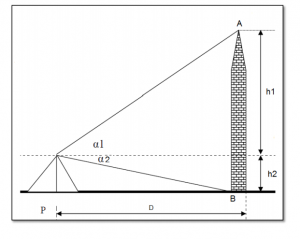# Measuring Height using Vertical Angle in Surveying

In this post, I am going to write about how to measure the height of an object using a vertical angle in surveying.

## Equipment used to measure the height of an object using a vertical angle

Theodolite, Leveling Stop, Tape or Chain, Pegs, Plumb bob

Sketch## Procedure to measure the height of an object using a vertical angle.(  Using theodolite)

1. Setup the instrument at station P.
3. Bring the line of collimation horizontal (vertical angle reading is zero).

4. Sight the top of the object (A), and read the vertical angle α1.
5. Sight the bottom of the object B, and read the vertical angle α2.
6. Measure the Horizontal distance between the instrument station and the object (D).
7. Calculate the height of the object as follow

h1 = D tan α1
h2 = D tan α2
The object height = h1 + h2

This is the method to find the height of an object using a vertical angle by using a theodolite instrument.

Open